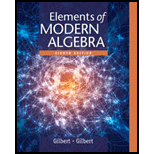# The solution of the equation [ 4 ] [ x ] = [ 5 ] in ℤ 13 , by finding [ a ] − 1 and using the result [ x ] = [ a ] − 1 [ b ] is the unique solution to the equation [ a ] [ x ] = [ b ] in ℤ n .### Elements Of Modern Algebra

8th Edition
Gilbert + 2 others
Publisher: Cengage Learning,
ISBN: 9781285463230### Elements Of Modern Algebra

8th Edition
Gilbert + 2 others
Publisher: Cengage Learning,
ISBN: 9781285463230

#### Solutions

Chapter 2.6, Problem 10E

(a)

To determine

## The solution of the equation [x]= in ℤ13, by finding [a]−1 and using the result [x]=[a]−1[b] is the unique solution to the equation [a][x]=[b] in ℤn.

(b)

To determine

(c)

To determine

(d)

To determine

(e)

To determine

(f)

To determine

(g)

To determine

(h)

To determine

### Want to see the full answer?

Check out a sample textbook solution.

### Want to see this answer and more?

Experts are waiting 24/7 to provide step-by-step solutions in as fast as 30 minutes!*

*Response times may vary by subject and question complexity. Median response time is 34 minutes for paid subscribers and may be longer for promotional offers.